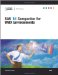# Chapter 8: Data Representation

## Numeric Variable Length and Precision in UNIX Environments

The default length of numeric variables in SAS data sets is 8 bytes. (You can control the length of SAS numeric variables with the LENGTH statement in the DATA step.)

The issue of numeric precision affects the return values of almost all SAS math functions and many numeric values returned from SAS procedures. Numeric values in SAS for UNIX are represented as IEEE double-precision floating-point numbers . The decimal precision of a full 8-byte number is effectively 15 decimal digits.

The following table specifies the significant digits and largest integer that can be stored exactly in SAS numeric variables.

Table 8.1: Significant Digits and Largest Integer by Length for SAS Variables under UNIX

Length in Bytes

Significant Digits Retained

Largest Integer Represented Exactly

3

3

8,192

4

6

2,097,152

5

8

536,870,912

6

11

137,438,953,472

7

13

35,184,372,088,832

8

15

9,007,199,254,740,992

When you are specifying variable lengths, keep in mind that variable length affects both the amount of memory used and the time required for I/O and arithmetic operations. See SAS Language Reference: Dictionary for more information about specifying variable lengths.

If you know that the value of a numeric variable will be between 0 and 100, you can use a length of 3 to store the number and thus save space in your data set. For example:

` data mydata;      length num 3;      ...  more SAS statements  ...   run; `

Numeric dummy variables (those whose only purpose is to hold 0 or 1) can be stored in a variable whose length is 3 bytes.

 Caution Use 3 bytes only for those variables with small values, preferably integers. If the value of a variable becomes large or has many significant digits, you might lose precision in arithmetic calculations when the length of a variable is less than 8 bytes.

For more information about specifying variable lengths and optimizing system performance, refer to SAS Language Reference: Concepts .SAS 9.1 Companion For Unix Enivronments
ISBN: 1590472101
EAN: 2147483647
Year: 2004
Pages: 185
Authors: SAS Institute